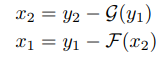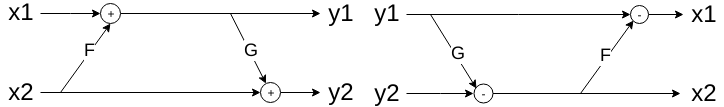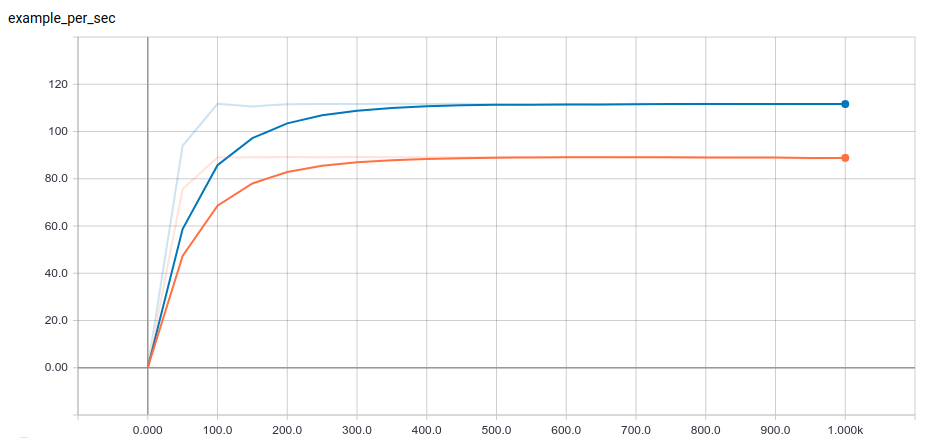Code with Eager Execution, Run with Graphs: Optimizing Your Code with RevNet as an Example
August 10, 2018
By Xuechen Li, Software Engineering Intern

#### Overview

Eager execution simplifies the model building experience in TensorFlow, whereas graph execution can provide optimizations that make models run faster with better memory efficiency. This blog post showcases how to write TensorFlow code so that models built using eager execution with the tf.keras API can be converted to graphs and eventually deployed on Cloud TPUs with the support of the tf.estimator API.

We use the Reversible Residual Network (RevNet, Gomez et al.) as an example. The following sections assume basic knowledge of convolutional neural networks and TensorFlow. The complete code of this article is located here (to ensure the code works properly in all settings, `tf-nightly` or `tf-nightly-gpu` is highly recommended).

#### RevNets

RevNets are like Residual Networks (ResNet, He et al.), except that they are reversible — — intermediate computation can be reconstructed given the output. One of the benefits of this is that we can save memory by reconstructing the activations as opposed to storing them all in memory during training (recall we need intermediate results to compute the gradient with respect to the input since the Chain Rule requires this). This allows us to fit larger batch sizes and train deeper models compared to regular backpropagation on traditional architectures. Concretely, this is achieved by using a set of cleverly constructed equations to define the network:where the top and bottom set of equations define the forward computation and its inverse respectively. Here x1 and x2 are inputs (split from the overall input x), y1 and y2 are outputs, and F and G are ConvNets. This enables us to exactly reconstruct the activations during backprop so that we don’t need to store them anymore during training.#### Define the Forward and Backward pass with tf.keras.Model

Supposing we have the class “ResidualInner” to instantiate functions F and G, we can define the reversible block by subclassing from `tf.keras.Model` and the forward pass by overriding the `call` method as in the above equations:
``````class Residual(tf.keras.Model):
def __init__(self, filters):
super(Residual, self).__init__()
self.f = ResidualInner(filters=filters, strides=(1, 1))
self.g = ResidualInner(filters=filters, strides=(1, 1))

def call(self, x, training=True):
x1, x2 = tf.split(x, num_or_size_splits=2, axis=self.axis)
f_x2 = self.f(x2, training=training)
y1 = f_x2 + x1
g_y1 = self.g(y1, training=training)
y2 = g_y1 + x2
return tf.concat([y1, y2], axis=self.axis)``````
The `training` argument here is used to determine the state of batch normalization. With eager execution enabled, the running averages of batch norm are updated automatically when `training=True`. When executing the equivalent graph, the batch norm updates need to be manually fetched with the method `get_updates_for`.

To build the memory-saving backward pass, we use `tf.GradientTape` as a context manager to trace gradients only where needed:
``````  def backward_grads(self, y, dy, training=True):
dy1, dy2 = dy
y1, y2 = y

gtape.watch(y1)
gy1 = self.g(y1, training=training)
x2 = y2 - gy1

ftape.watch(x2)
fx2 = self.f(x2, training=training)
x1 = y1 - fx2

x = x1, x2
dx = dx1, dx2

The exact set of gradient computation can be found in Algorithm 1 of the paper (we simplified in our code the intermediate steps that use variable z1). The algorithm is designed so that within each reversible block, gradients with respect to the input and model variables are computed along with reconstructing the input, given both the output and the gradient of the loss with respect to the output. Calling `tape.gradient(y, x)` computes the gradient of y with respect to x. We can also use the argument `output_gradients` to explicitly apply the chain rule.

#### Eager Execution for Faster Prototyping

One of the obvious benefits of prototyping with eager execution is that it is imperative. We can obtain results immediately as opposed to building a graph first and then initializing a session to run.

For instance, we validate our model by comparing the reversible backprop gradients with the gradients computed by regular backprop:
``````block = Residual()
x = tf.random_normal(shape=(N, C, H, W))
dy = tf.random_normal(shape=(N, C, H, W))
tape.watch(x)
y = block(x)

dx, _ = block.backward_grads(x, y, dy)

# Check whether the difference is below a certain threshold
thres = 1e-6
diff_abs = tf.reshape(abs(dx - dx_true), [-1])
assert all(diff_abs < thres)``````
In the above snippet, `dx_true` is the gradient returned by regular backprop, whereas `dx` is the gradient returned by our implementation of reversible backprop. Eager execution integrates with native Python so that functions like `all` and `abs` can be directly applied to Tensors.

#### Store and Load Checkpoints with tf.train.Checkpoint

To ensure saving and loading checkpoints work with both eager and graph execution, the TensorFlow team recommends using `tf.train.Checkpoint` API.

In order to store a model, we create an instance of `tf.train.Checkpoint` with all the objects we want to store. This may include our model, optimizers we use, the learning rate schedule, and the global step:
``````checkpoint = tf.train.Checkpoint(model=model, optimizer=optimizer,
learning_rate=learning_rate, global_step=global_step)``````
We can save and restore a particular trained instance as follows:
``````checkpoint.save(file_prefix)
checkpoint.restore(save_path)``````

#### Boost Eager Execution Performance with tf.contrib.eager.defun

Eager execution can sometimes be slower than executing the equivalent graph due to overheads of interpreting Python code. This performance gap can be bridged by compiling Python functions composed of TensorFlow operations into callable TensorFlow graphs via `tf.contrib.eager.defun`. When training a deep learning model, there are typically three major places where we can apply `tf.contrib.eager.defun`: 1) the forward computation, 2) the backward computation for the gradients, and 3) the application of gradients to variables. As an example, we can defun the forward pass and the gradient computation as follows:
``````tfe = tf.contrib.eager
model.call = tfe.defun(model.call)
To defun the optimizer’s apply gradients step, we need to wrap it inside another function:
``````def apply_gradients(optimizer, gradients, variables, global_step=None):
`tf.contrib.eager.defun` is under active development, and applying it is an evolving technique; for more information, consult its docstring.

Wrapping a Python function with `tf.contrib.eager.defun` causes the TensorFlow API calls in the Python function to build a graph instead of immediately executing operations, enabling whole program optimizations. Not all Python functions can be successfully converted to an equivalent graph, particularly those with dynamic control flow (e.g., an `if` or `while` on `Tensor` `contents`). `tf.contrib.autograph` is a related tool that increases the surface area of Python code that can be converted to a TensorFlow graph. As of August 2018, integration of autograph with defun was in progress.

#### Build Input Pipeline with TFRecords and tf.data.Dataset

Eager execution is compatible with the `tf.data.Dataset` API. We can read a TFRecords file:
``````dataset = tf.data.TFRecordDataset(filename)
dataset = dataset.repeat(epochs).map(parser).batch(batch_size)``````
To improve performance we can also use the `prefetch` function and adjust `num_parallel_calls`.

Looping over this dataset in eager execution is simple given that the dataset consists of image, label pairs. In this case, we don’t even need to explicitly define an iterator:
``````for image, label in dataset:
logits = model(image, training=True)
...``````

#### Wrap Keras Models in Estimators and Execute as Graphs

Since the `tf.keras` API also supports graph building, the same model built using eager execution can also be used as a graph-construction function provided to an `Estimator`, with few changes to the code. To modify the RevNet example built in eager execution, we need only wrap the keras model in a `model_fn` and use it according to the `tf.estimator` API.
``````def model_fn(features, labels, mode, params):
model = RevNet(params["hyperparameters"])
if mode == tf.estimator.ModeKeys.TRAIN:
optimizer = tf.train.MomentumOptimizer(learning_rate, momentum)
logits, saved_hidden = model(features, training=True)
return tf.estimator.EstimatorSpec(mode=mode, loss=loss, train_op=train_op)``````
The `input_fn` required by the `tf.estimator` API can be defined as usual using the `tf.data` API, reading from TFRecords.

#### Wrap Keras Models in TPU Estimators for Cloud TPU Training

Wrapping the model and input pipeline in an `Estimator` allows the model to run on Cloud TPUs.

The steps needed are:
1. Set up Cloud TPU specific configurations
2. Switch from `tf.estimator.Estimator` to `tf.contrib.tpu.TPUEstimator`
3. Wrap the usual optimizers in `tf.contrib.tpu.CrossShardOptimizer`
For a concrete demonstration, check out the TPU estimator script in the RevNet example folder. We expect the process of enabling a Keras model to run on TPUs to be further simplified with `tf.contrib.tpu.keras_to_tpu_model` in the future.

#### Optional: Model Performance

`tf.GradientTape`, coupled with a simplification of the gradient computation that obviates the need for an extra forward pass, allows us to implement RevNet’s reversible backprop with a computational overhead of just 25% compared to regular backprop.

The blue and orange curves represent examples/sec for usual backprop and reversible backprop respectively as the global step increases. The plot is from RevNet-104 trained on mock ImageNet data with a batch size of 32 on a single Tesla P100.To verify memory savings, we plot memory usage as training progresses. The blue and black curves are regular and reversible backprop respectively. The plot records 100 iterations of RevNet-104 graph-mode training on mock ImageNet data with a batch size of 128. The plot was generated by mprof while training was performed on CPU so that we can train with the same batch size in regular backprop.#### Conclusion

With RevNet as an example, we have demonstrated how to quickly prototype machine learning models with eager execution and the `tf.keras` API. This simplifies the model building experience and moreover, with little extra effort, we can convert our model to estimators and deploy them on Cloud TPUs for high performance. You can find the complete code for this article here. Also, make sure to checkout other examples with eager execution.
Next postBy Xuechen Li, Software Engineering Intern
OverviewEager execution simplifies the model building experience in TensorFlow, whereas graph execution can provide optimizations that make models run faster with better memory efficiency. This blog post showcases how to write TensorFlow code so that models built using eager execution with the tf.keras API can be converted to graphs and eventually deploye…